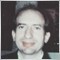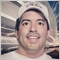# Range not behaving as expected - page 224281

 When drawing pivots, there is no need to redraw bar zero as the value doesn't change. Unlike a moving average. ```        #define REDRAW_BAR_ZERO false           // Don't redraw Bar zero.         return rates_total - MathMax(REDRAW_BAR_ZERO, iLast); ```24281

Here's how to do the drawing in groups (when the terminal becomes unresponsive on startup.)
#define LAST 0
const int   LB_MA    = LengthMMA-1;
int      iMA     = rates_total - 1 - MathMax(LB_MA, prev_calculated);
int      iLast   = MathMax(LAST, iMA - 2000);        // Do in groups
for(; iMA >= iLast; --iMA){
:
}  // iMA
//----
#define REDRAW_BAR_ZERO true     // Redraw Bar zero.
return rates_total - MathMax(REDRAW_BAR_ZERO, iLast);24281

 The last line above can be optimized (and must be for MTF version.) return   rates_total - REDRAW_BAR_ZERO - iLast; For multi-timeframe indicators, there are changes. // Input(s)extern ENUM_TIMEFRAMES TimeFrame    = PERIOD_CURRENT;//+------------------------------------------------------------------+//| Custom indicator initialization function                         |//+------------------------------------------------------------------+int OnInit(){   if(TimeFrame == PERIOD_CURRENT)  TimeFrame   = (ENUM_TIMEFRAMES) _Period;   if(TimeFrame < _Period) return INIT_PARAMETERS_INCORRECT; The look back must adjusted when the look back is from a higher TF.Don't mix apples and oranges const int   lookback    = TimeFrame/_Period; // * 1   int      iBar  =  Rates_total - 1 - MathMax(lookback, prev_calculated);   #define LAST 0   int      iLast = MathMax(LAST, iBar - 2000);   // Do in groups   for(; iBar >= iLast; --iBar){      int       iTF = iBarShift(NULL, TimeFrame, Time[iBar]);      double   hiCur    = iHigh(NULL, TimeFrame, iTF),   //High[iBar],                 hiPre    = iHigh(NULL, TimeFrame, iTF+1); //High[iBar+1];      : Redraw bar zero of the higher TF to get the stair step not just the chart's bar zero.(See images Multi TimeFrame Moving Average or Moving Average Ex from the codebase) #define  REDRAW_BAR_ZERO true       // Redraw Bar zero.   iLast = iBarShift(NULL, 0, Time[iLast] - Time[iLast] % (TimeFrame * 60));   return   rates_total - REDRAW_BAR_ZERO - iLast;24281

 Rather then computing iLast (as I did above,) I could "back up" to the last non-zero TimeFrame bar for the stair step effect. Note the dropping the minus one from iBar calculation and changing the for to a while loop, simplify things. ```   const int   lookback    = TimeFrame/_Period; // * 1    int      iBar  = Rates_total - MathMax(lookback, prev_calculated);    int      iLast = iBar;    while(iBar > 0){  --iBar;       int       iTF = iBarShift(NULL, TimeFrame, Time[iBar]);       if(iTF != 0) iLast = iBar;       double   hiCur    = iHigh(NULL, TimeFrame, iTF),   //High[iBar],         :    }    #define  REDRAW_BAR_LAST false      // Don't redraw TF Bar one.    return   rates_total - REDRAW_BAR_LAST - iLast;```725

The lookback limit works very well for me in MT5 to limit the number of bars drawn by the custom indicator and save resources. However, when a new chart is downloading new data, I can sometimes witness garbled data appearing past bar 1000, which was never filled in by the custom indicator. I know that the indicator didn't fill in those values, as it never looked past 1000 bars, and it cancels if it cannot access any price data yet (downloading a new chart). Where does the garbled data come from? Of course, I found an easy way to fix this, which was to fill all 50,000 bars on the chart with EMPTY_VALUE. That solves the problem. The fix looks like this:

if (prev_calculated==0) for (j=0; j<rates_total; j++) indicatorBuffer[j]=EMPTY_VALUE;

This works, and is fast because it only has to loop once on the first tick, or only a few times when new data is being downloaded to a new chart. However, it looks a bit messy and has to loop 50,000 bars, or even 500,000 if that many are on the chart. Is there a nicer, cleaner solution for this?24281

Victor Epand: I know that the indicator didn't fill in those values, as it never looked past 1000 bars,
1. >Yes it did. It filled them when there were less than 1K bars on chart. Then newer history came in. Now there are 2K bars with values in the older part.

Always set buffer values, do not assume that they are empty values.

```  while(iBar > 0){  --iBar;
buffer[iBar] = EMPTY_VALUE;
:```

2. I never liked those arbitrary look back limits. I use the attached file to process as many bars as possible in 1.5 seconds and then continue periodically(either by time or by tick,) until all bars are done.
Files:181

Victor Epand:

The lookback limit works very well for me in MT5 to limit the number of bars drawn by the custom indicator and save resources. However, when a new chart is downloading new data, I can sometimes witness garbled data appearing past bar 1000, which was never filled in by the custom indicator. I know that the indicator didn't fill in those values, as it never looked past 1000 bars, and it cancels if it cannot access any price data yet (downloading a new chart). Where does the garbled data come from? Of course, I found an easy way to fix this, which was to fill all 50,000 bars on the chart with EMPTY_VALUE. That solves the problem. The fix looks like this:

if (prev_calculated==0) for (j=0; j<rates_total; j++) indicatorBuffer[j]=EMPTY_VALUE;

This works, and is fast because it only has to loop once on the first tick, or only a few times when new data is being downloaded to a new chart. However, it looks a bit messy and has to loop 50,000 bars, or even 500,000 if that many are on the chart. Is there a nicer, cleaner solution for this?

Is there a nicer, cleaner solution for this?

Yes, there is a cleaner (and extremely faster) solution to do that, and without using a loop:

```   if(prev_calculated == 0) {
ArrayInitialize(indicatorBuffer,EMPTY_VALUE);
}
```1467

The_Rezzer:

Goodmo

To get the values of HA you have to immitate the MT way

If you use Array Notsetasserie, your last bar is not 0 zéro bar, but rates_total
``` if(prev_calculated>1)
{
pos=prev_calculated-1;
}
else                     // first bar is bar 0    <=> prev_caculated == 0
{
//--- set first candle
if(open<close)
{
ExtLowHighBuffer=low;
ExtHighLowBuffer=high;
}
else
{
ExtLowHighBuffer=high;
ExtHighLowBuffer=low;
}
ExtOpenBuffer=open;
ExtCloseBuffer=close;
//---
pos=1;
}                  //end first bar 0

// pos == 1 only once, the first time,  then :
//  pos=prev_calculated-1;   <=> for(i=prev_calculated-1; i<rates_total; i++) ==  main loop
// only one bar is recalculated at each tick
//--- main loop of calculations
for(i=pos; i<rates_total; i++)
{
haOpen=(ExtOpenBuffer[i-1]+ExtCloseBuffer[i-1])/2;
haClose=(open[i]+high[i]+low[i]+close[i])/4;
haHigh=MathMax(high[i],MathMax(haOpen,haClose));
haLow=MathMin(low[i],MathMin(haOpen,haClose));
if(haOpen<haClose)
{
ExtLowHighBuffer[i]=haLow;
ExtHighLowBuffer[i]=haHigh;
}
else
{
ExtLowHighBuffer[i]=haHigh;
ExtHighLowBuffer[i]=haLow;
}
ExtOpenBuffer[i]=haOpen;
ExtCloseBuffer[i]=haClose;
}```

now you have your values for HA and if you want to do further caculation you have to use this loop:

`for(i=prev_calculated-1; i<rates_total; i++)`

i am not sure that ArrayMaximum will work in this case24281

#14 can be simplified

Regular indicator:

```while(iBar > 0){ --iBar;
⋮
}
return rates_total - (iBar + 1); // Redraw iBar.```

MTF indicator:

```      while(mtf_shift(iBar) == 0)   ++iBar;  // Redraw higher TF bar zero.
return rates_total - iBar;
///
INDEX    mtf_shift(INDEX iBar){
return   iBarShift(_Symbol, SourceTF, Time[iBar]);
}
```